## 1 Introduction

Quite recently Bauer et al.  have investigated a generalization of Inoue’s construction  of the classical Burniat surfaces . These surfaces are minimal, of general type and have invariants $$p_g=q=0,1,2$$ or 3.

Recall that the Chow group $$\mathop {\mathsf {CH}}\nolimits _k$$ is said to be “trivial”, if the natural cycle class map $$\mathop {\mathsf {CH}}\nolimits _k \hookrightarrow H_{2k}$$ is injective. The kernel of the cycle class map $$\mathop {\mathsf {CH}}\nolimits _k^{\mathrm{hom}}$$ can be investigated by means of the Abel–Jacobi map $$\mathop {\mathsf {CH}}\nolimits _k^{\mathrm{hom}} \rightarrow J^k$$, where the target is the kth intermediate Jacobian. Its kernel is denoted $$\mathop {\mathsf {CH}}\nolimits _k^{\mathrm{AJ}}$$. If this vanishes, this has strong consequences. For instance for surfaces this implies $$p_g=0$$ and the Albanese map is an isomorphism up to torsion. The converse is Bloch’s conjecture . In a follow-up study  this conjecture has been verified for the generalized Burniat surfaces, i.e $$\mathop {\mathsf {CH}}\nolimits _0^{\mathrm{hom}}=0$$.

These generalized Burniat surfaces $$Y=X/G$$ are all quotients of X by a freely acting abelian group $$G\simeq (\mathbf {Z}/2\mathbf {Z})^3$$ and where (XG) is a so called Burniat hypersurface pair (XG): X is a hypersurface in a product A of three elliptic curves having at most nodes as singularities and G is an abelian group acting freely on A and leaving X invariant. The surface X is also called Burniat hypersurface. These come in 16 families, enumerated $$\mathscr {S}_1$$$$\mathscr {S}_{16}$$. The classical Burniat surface belongs to the 4-parameter family $$\mathscr {S}_2$$. Also the family $$\mathscr {S}_1$$ is 4-dimensional. The remaining families have only 3 parameters coming from varying the elliptic curve. This implies that the equation of X in these cases is uniquely determined, contrary to the first two where there is a pencil of hypersurfaces invariant under G. The surfaces Y have at most nodal singularities. For simplicity I assume in this note that Y, and hence X is smooth, which is generically the case. However, none of the arguments is influenced by the presence of nodal singularities.

In  it has been remarked that the main theorem of loc. cit. yields the Bloch conjecture for the classical Burniat surfaces. The goal of this paper is to apply the same methods to all Burniat hypersurfaces. In particular, one obtains a short proof of the Bloch conjecture in the appropriate cases.

To state the result, let me recall that the Chow motiveFootnote 1 $$\mathsf h(X)$$ is the pair $$(X,\Delta )$$ where $$\Delta \subset X\times X$$ is the diagonal considered as a (degree 0) self-correspondence of X. As a self-correspondence it is an idempotent in the ring $$\mathop {\mathrm{Corr}}\nolimits ^0(X)$$ of degree 0 self-correspondences. If a finite group G acts on X, any character $$\chi$$ of the group defines an idempotent

\begin{aligned} \pi _\chi = \frac{1}{|G|} \sum _{g\in G} \chi (g) \Gamma _g \in \mathop {\mathrm{Corr}}\nolimits ^0(X), \end{aligned}

where $$\Gamma _g$$ is the graph of the action of g on X. The pair $$(X,\pi _\chi )$$ is the motive canonically associated to the character $$\chi$$. Note that the trivial character gives the motive $$\mathsf h(X/G)$$ of the Burniat hypersurface. The main result now reads as follows:

### Theorem

(=Theorem 4.2) With $$i:X\hookrightarrow A$$ the inclusion, let (XG) be a Burniat hypersurface pair as before and let $$Y=X/G$$ be the corresponding generalized Burniat surface. Consider the one-dimensional space $$H^0(\Omega ^3_A)$$ as a G-representation space, i.e. as a character $$\chi _A$$. Then

1. (1)

For the families $$\mathscr {S}_1,\mathscr {S}_2$$ the involution $$j=\iota _1\iota _2\iota _3$$ belongs to G and the motives $$\mathsf h(X/j)$$ and $$\mathsf h(Y)$$ are finite dimensional.

2. (2)

For all other families, the motive $$(X,\pi _{\chi _A})$$ is finite-dimensional. For the families $$\mathscr {S}_3$$,$$\mathscr {S}_4$$, $$\mathscr {S}_{11},\mathscr {S}_{12},\mathscr {S}_{16}$$ this motive is just $$\mathsf h(Y)$$.

3. (3)

The Bloch conjecture holds for the families $$\mathscr {S}_1$$$$\mathscr {S}_4$$. In the remaining cases a variant of Bloch’s conjecture holds, namelyFootnote 2 $$\ker (i_* :\mathop {\mathsf {CH}}\nolimits _0^\mathrm{AJ}(X)^{\chi _A} \rightarrow \mathop {\mathsf {CH}}\nolimits _0^{\mathrm{AJ}}(A)^{\chi _A} )=0$$. For the families $$\mathscr {S}_{11},\mathscr {S}_{12}$$ and $$\mathscr {S}_{16}$$ this means that $$\ker (i_* :\mathop {\mathsf {CH}}\nolimits _0(X) \rightarrow \mathop {\mathsf {CH}}\nolimits _0(A) )=0$$.Footnote 3

As shown in  the families $$\mathscr {S}_{11}$$ and $$\mathscr {S}_{12}$$ give two divisors in a 4-dimensional component of the Gieseker moduli space.

The above method applies also to the surfaces in this component, the so-called Sicilian surfaces so that the result for $$\mathscr {S}_{11}$$ and $$\mathscr {S}_{12}$$ is valid for these as well. See Remark 4.3.

## 2 Preliminaries

### 2.1 Motives

A degree k (Chow) correspondences from a smooth projective variety X to a smooth projective variety Y is a cycle class

\begin{aligned} \mathop {\mathrm{Corr}}\nolimits ^k(X,Y){:}{=} \mathop {\mathsf {CH}}\nolimits ^{\dim X+k}(X\times Y). \end{aligned}

A correspondence of degree k induces a morphism on Chow groups of the same degree and on cohomology groups (of double the degree). Correspondences can be composed and these give the morphisms in the category of Chow motives. Let me elaborate briefly on this but refer to  for more details.

Precisely, an effective Chow motive consists of a pair (Xp) with X a smooth projective variety and p a degree zero correspondence which is a projector, i.e., $$p^2=p$$. Morphism between motives are induced by degree zero correspondences compatible with projectors. This procedure defines the category of effective Chow motives. Every smooth projective variety X defines a motive

\begin{aligned} {\mathsf h}(X)= (X,\Delta ),\quad \Delta \in \mathop {\mathsf {CH}}\nolimits ^{\dim X}( X\times X) \text { the class of the diagonal} \end{aligned}

and a morphism $$f:X\rightarrow Y$$ between smooth projective varieties defines a morphism $${\mathsf h}(Y) \rightarrow {\mathsf h}(X)$$ given by the transpose of the graph of f.

One can also use correspondences of arbitrary degrees provided one uses triples (Xpk) where p is again a projector, but a morphism $$f: (X,p,k) \rightarrow (Y,q,\ell )$$ is a correspondence of degree $$\ell -k$$ compatible with projectors. Such triples define the category of Chow motives. It should be recalled that motives, like varieties have their Chow groups and cohomology groups: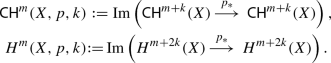Kimura  has introduced the concept finite-dimensionality for motives. If the motive of a surface S is finite-dimensional, then the Bloch conjecture holds for any submotive M of S with $$h^{2,0}(M)=0$$. This is the motivation for considering the variable cohomology. In  it is shown that there is indeed a submotive of S whose cohomology is the variable motive.

### 2.2 A criterion for finite dimensional motives

The general situation of  concerns smooth d-dimensional complete intersections X inside a smooth projective manifold M of dimension $$d+r$$ for which Lefschetz’ conjecture B(M) holds. This conjecture is known to hold for projective space and for abelian varieties and so in particular for the situation in this note.

Recall also (see e.g. [5, Ch. 3.2]) that in this situation, with $$i:X\hookrightarrow M$$ the inclusion, the fixed and variable cohomology is defined as follows.

\begin{aligned} \begin{aligned} H^d_{\mathrm{fix}}(X)&={\text {Im}}( i^* : H^{d}(M) \rightarrow H^d(X)),\\ H^d_{\mathrm{var}}(X)&= \ker (i_*:H^{d} (X)\rightarrow H^{d+2r}(M)), \end{aligned} \end{aligned}

and that one has a direct sum decomposition

\begin{aligned} H^d(X)= H_{\mathrm{fix}}^d(X) \oplus H_{\mathrm{var}}^d(X), \end{aligned}

which is orthogonal with respect to the intersection product. In  I explained that validity of B(M) implies the existence of a motive $$(X,\pi ^{\mathrm{var}})$$ such that $$\pi ^{\mathrm{var}}$$ induces projection onto variable cohomology.

The main input is the special case of [9, Thm. 6.5 and Cor. 6.6] for surfaces inside a threefold. It reads as follows.

### Theorem 2.1

Let M be a smooth projective threefold on which a finite abelian group G acts. Let $${\mathscr {L}}$$ be line bundle with G-action, $$X\subset M$$ a G-invariant section and $$\chi$$ a character of G. Suppose that

1. (1)

the conjecture B(M) holds;

2. (2)

the sections of $$H^0(M,{\mathscr {L}})^G$$ separates orbits;

3. (3)

all characters of G appear in $$\mathop {\mathrm{End}}\nolimits (H^{2}_{\mathrm{var}}(X))$$;

4. (4)

the Chow motive $$(M,\pi _\chi )$$ is finite-dimensional;

5. (5)

$$0 \not = H^2 _{\mathrm{var}}(X)$$ and $$H^2_{\mathrm{var}} (X)^{\chi }$$ is pure of Hodge type (1, 1).

Then $$\mathop {\mathsf {CH}}\nolimits _0^{\mathrm{var}}(X)^{\chi }=0$$, and the motive $$(X,\pi _\chi )$$ is finite-dimensional.

### 2.3 Elliptic curves

Let me recall the relevant facts about theta functions on an elliptic curve E with period lattice $$\Lambda$$ generated by 1 and $$\tau \in \mathfrak h$$. Points in the elliptic curve referred to by the standard coordinate $$z\in \mathbf {C}$$ and the corresponding line bundle by $${\mathscr {L}}_z$$. It is the bundle with $$H^0(E,{\mathscr {L}}_z)= \mathbf {C}\vartheta _z$$, $$\vartheta _z$$ a theta-function with simple zeros in the points $$z + \Lambda$$ only. Let $$t_u : z \mapsto z+u$$ be a translation of E. Then $${\mathscr {L}}_z \simeq t_z^* {\mathscr {L}}_0$$. If one takes for z one of the four two-torsion points $$\epsilon \in \{0, \frac{1}{2},\frac{1}{2}\tau , \frac{1}{2}+\frac{1}{2}\tau \}$$ of E, the corresponding line bundles $${\mathscr {L}}_\epsilon$$ have the four classical theta functions $$\vartheta _1, \vartheta _2,\vartheta _3, \vartheta _4$$ respectively as sections. See e.g. [6, Appendix A, Table 16 ] for the definitions.

Set

\begin{aligned} M_E{:}{=} H^0(E,{\mathscr {L}}_0^2). \end{aligned}

### Lemma 2.2

1. (i)

The bundle $${\mathscr {L}}_0^2$$ is a symmetric line bundle and all its sections are symmetric.

2. (ii)

The translations $$t_\epsilon$$ define a faithful action of $$(\mathbf {Z}/2\mathbf {Z})^2$$ on $${\mathscr {L}}_0^2$$.

3. (iii)

The character decomposition of $$M_E$$ for this action is $$(+-)\oplus (-+)$$.

### Proof

1. (i)

is clear.

2. (ii)

Since $${\mathscr {L}}_\epsilon ^2 \simeq {\mathscr {L}}_0^2$$ for all two-torsion points $$\epsilon$$, the functions $$\vartheta _j^2$$ define sections of the same bundle $${\mathscr {L}}_0^2$$. The sections $$\vartheta _j^2$$, $$j=1,2,3,4$$ are characterized by having a double zero at exactly one of the four 2-torsion points. This shows in particular that the action of the group $$\{ t_\epsilon , \epsilon \text { a 2-torsion point}\}$$ is faithful on $$M_E$$.

3. (iii)

It follows that there is a basis of two sections of $${\mathscr {L}}_0^2$$ consisting of simultaneous eigenvectors for this action. Since the action is faithful, the character decomposition must be $$(+-), (-+)$$.

$$\square$$

## 3 Surfaces inside abelian threefolds invariant under involutions

### 3.1 Products of three elliptic curves

Consider the abelian threefold

\begin{aligned} A{:}{=} E_1 \times E_2 \times E_3 , \quad E_\alpha = \mathbf {C}/\Lambda _\alpha , \, \text { with } \quad \Lambda _\alpha =\mathbf {Z}\oplus \mathbf {Z}\tau _\alpha ,\, \alpha =1,2,3. \end{aligned}

Using for a fixed elliptic curve $$E=\mathbf {C}\mathbf {Z}\oplus \tau \mathbf {Z}$$ the involutions

\begin{aligned} \iota _E : z\mapsto -z, \quad t_E : z\mapsto - z+{\frac{1}{2}} ,\quad {\tau }_E : z \mapsto - z+ {\frac{1}{2}\tau }, \end{aligned}

we obtain three involutions on A

\begin{aligned} \begin{aligned} \iota _\alpha&=\iota _{E_\alpha } \\ \iota _{\alpha \beta }&= t_{E_\alpha } t_{E_\beta },\\ \iota _{123}&= \tau _{E_1}\tau _{E_2}\tau _{E_3}. \end{aligned} \end{aligned}
(1)

and we consider the group $$(\mathbf {Z}/2\mathbf {Z})^6$$ operating on A as

\begin{aligned} G_0 {:}{=} \langle \, \iota _1, \iota _2, \iota _3 ,\iota _{12} , \iota _{13}, \iota _{123} \, \rangle . \end{aligned}

### Lemma 3.1

The action of $$G_0$$ on holomorphic 1-forms of A is given by

Form

$$\iota _1$$

$$\iota _2$$

$$\iota _3$$

$$\iota _{12}$$

$$\iota _{13}$$

$$\iota _{23}$$

$$\iota _{123}$$

$$dz_1$$

$$-$$

$$+$$

$$+$$

$$+$$

$$-$$

$$dz_2$$

$$+$$

$$+$$

$$-$$

$$+$$

$$-$$

$$-$$

$$dz_3$$

$$+$$

$$+$$

$$+$$

$$-$$

$$-$$

Consider now the symmetric line bundle $${\mathscr {L}}_A^2$$ where

\begin{aligned} {\mathscr {L}}_A:={\mathscr {O}}_{E_1}( {\mathscr {L}}_0) \boxtimes {\mathscr {O}}_{E_2}( {\mathscr {L}}_0) \boxtimes {\mathscr {O}}_{E_3}( {\mathscr {L}}_0), \end{aligned}

and set

\begin{aligned} H^0({\mathscr {L}}_A^2) = M_{E_1} \boxtimes M_{E_3} \boxtimes M_{E_3}. \end{aligned}

By Lemma 2.2 this is a representation space for $$G_0$$ which admits a basis of simultaneous eigenvectors. If {$$\theta ^1_{E_j}, \theta ^2_{E_j}\}$$, denotes the basis of Lemma 2.2, for $$M_{E_j}$$, $$j=1,2,3$$, their products give 8 basis vectors as follows.

\begin{aligned} \theta _{j_1j_2j_3}= \theta _{E_1}^{j_1} \cdot \theta _{E_2}^{j_2} \cdot \theta _{E_3}^{j_3},\quad j_k\in {1,2}. \end{aligned}

The next result is a consequence.

### Lemma 3.2

The space $$H^0({\mathscr {L}}^2_A)$$ is the $$G_0$$-representation space which on the basis $$\{ \theta _{j_1j_2j_3}\}$$, $$j_1,j_2,j_3\in \{1,2\}$$, is given as follows

Element

$$\iota _1$$

$$\iota _2$$

$$\iota _3$$

$$\iota _{12}$$

$$\iota _{13}$$

$$\iota _{23}$$

$$\iota _{123}$$

$$\theta _{111}$$

$$+$$

$$+$$

$$+$$

$$+$$

$$+$$

$$+$$

$$\theta _{211}$$

$$+$$

$$+$$

$$+$$

$$-$$

$$+$$

$$+$$

$$\theta _{121}$$

$$+$$

$$+$$

$$+$$

$$+$$

$$+$$

$$\theta _{112}$$

$$+$$

$$+$$

$$+$$

$$+$$

$$+$$

$$\theta _{1\, 2\, 2}$$

$$+$$

$$+$$

$$+$$

$$-$$

$$+$$

$$\theta _{2\, 1\, 2 }$$

$$+$$

$$+$$

$$+$$

$$-$$

$$+$$

$$\theta _{221}$$

$$+$$

$$+$$

$$+$$

$$+$$

$$\theta _{222}$$

$$+$$

$$+$$

$$+$$

$$+$$

$$+$$

$$+$$

$$+$$

### 3.2 Hypersurfaces of abelian threefolds and involutions

Let A be an abelian variety of dimension three and $${\mathscr {L}}$$ a principal polarization so that $${\mathscr {L}}^3=3! =6$$ and let $$i: X\hookrightarrow A$$ be a smooth surface given by a section of of $${\mathscr {L}}^{\otimes 2}$$. The Lefschetz’s hyperplane theorem gives: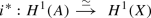(2)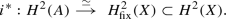(3)

### Lemma 3.3

Suppose that $$\iota :A\rightarrow A$$ is an involution which acts on $$H^0(\Omega ^1_A)$$ with p eigenvalues 1 and $$n=3-p$$ eigenvalues $$-1$$. Suppose also that $$\iota$$ preserves X and acts without fixed points on X. Then we have

\begin{aligned} \mathop {\mathrm{Tr}}\nolimits (\iota ) |H^2_{\mathrm{var}}(X) = -29 +8p(4-p) = {\left\{ \begin{array}{ll} -\,29 &{} \text {for } \quad p=0\\ -\,5 &{} \text {for }\quad p=1 \\ \,\,3 &{} \text {for }\quad p=2 \\ \,-5 &{} \text {for } \quad p=3. \end{array}\right. } \end{aligned}

### Proof

The assumption implies that

\begin{aligned} \mathop {\mathrm{Tr}}\nolimits (\iota )|H^1(A) = 4p - 6= {\left\{ \begin{array}{ll} -\,6 &{} \text {for } \quad p=0\\ -\,2 &{} \text {for } \quad p=1 \\ \, 2 &{}\text {for }\quad p=2\\ \, 6&{} \text {for } \quad p=3, \end{array}\right. } \end{aligned}

and

\begin{aligned} \mathop {\mathrm{Tr}}\nolimits (\iota )| H^2(A)= 8p(p-3)+15= {\left\{ \begin{array}{ll} 15 &{} \text {for }\quad p=0\\ -\,1 &{} \text {for }\quad p=1 \\ {-\,1}&{} \text {for } \quad p=2 \\ \, 15&{} \text {for }\quad p=3. \end{array}\right. } \end{aligned}

If $$\iota$$ preserves X and acts without fixed points on X, Lefschetz’ fixed point theorem gives $$0= 2 - 2 \mathop {\mathrm{Tr}}\nolimits (\iota ) | H^1(X) + \mathop {\mathrm{Tr}}\nolimits (\iota ) | H^2(X)= 2 - 2\mathop {\mathrm{Tr}}\nolimits (\iota ) | H^1(A) + \mathop {\mathrm{Tr}}\nolimits (\iota ) | H^2(A) + \mathop {\mathrm{Tr}}\nolimits (\iota ) |H^2_\mathrm{var}(X) ,$$ and so the above calculation immediately gives the desired result. $$\square$$

In order to calculate the invariants on X, let me first consider the holomorphic two-forms in detail.

### Lemma 3.4

1. 1.

One has

\begin{aligned} h^{0,2}_{\mathrm{var}} (X) = 7,\quad h^{0,2}_{\mathrm{fix}} (X)= 3. \end{aligned}
2. 2.

If $$X=\{\theta _0=0\}$$, the variable holomorphic 2-forms are the Poincaré-residues along X of the meromorphic 3-forms on A given by expressions of the form

\begin{aligned} \frac{\theta }{\theta _0} dz_1\wedge dz_2\wedge dz_3 \end{aligned}

with $$\theta$$ a theta-function on A corresponding to a section of $${\mathscr {L}}^{\otimes 2}$$, and where $$z_1,z_2,z_3$$ are holomorphic coordinates on $$\mathbf {C}^3$$.

3. 3.

Suppose $$\iota$$ acts with the character $$\epsilon \in \{\pm 1\}$$ on holomorphic three forms. Let (pn) be the dimensions of the invariant, resp. anti-invariant sections of $${\mathscr {L}}^{\otimes 2}$$. Then $$\dim H^{2,0}_{\mathrm{var,{\epsilon }}}(X)= p-1$$ if $$\epsilon =1$$ and $$=p$$ otherwise.

### Proof

Consider the Poincaré residue sequence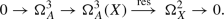In cohomology this gives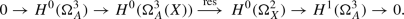(4)

One sees that image of the residue map coincides with $$\ker {\left( H^0(\Omega ^2_X) \rightarrow H^1(\Omega ^3_A)\right) }$$, which is the (2, 0)-part of the variable cohomology, by definition equal to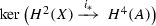. Since $$H^0(\Omega ^3_A(X))= H^0({\mathscr {L}}^{\otimes 2})$$ the assertion 1. follows.

1. 2.

This is clear.

2. 3.

This follows directly from (4). $$\square$$

### Corollary 3.5

The invariants of X are as follows.

$$b_1$$

$$b_2^{\mathrm{var}}=(h^{2,0}_{\mathrm{var}},h^{1,1} _{\mathrm{var}},h^{0,2} _{\mathrm{var}} )$$

$$b_2^{\mathrm{fix}}=(h^{2,0}_{\mathrm{fix}},h^{1,1} _{\mathrm{fix}},h^{0,2} _{\mathrm{fix}} )$$

6

$$43=(7,29,7)$$

$$15=(3,9,3)$$

### Proof

Equation (2) gives $$b_1(X)=b_1(A)=6$$. To calculate $$b_2(X)$$ we observe that $$c_1(X)= -2 {\mathscr {L}}|_X$$ and $$c_2(X)= 4 {\mathscr {L}}^2|_X$$ so that

\begin{aligned} c_1^2(X)=c_2(X)=4 {\mathscr {L}}^2|_X=8 {\mathscr {L}}^3 =48. \end{aligned}

Since $$c_2(X)= e(X)=2- 2b_1(X)+ b_2(X)=48$$, it follows that $$b_2(X)= 58$$. By (3) one has $$b_2^{\mathrm{fix}}(X)= b_2(A)=15$$ and so $$b_2^{\mathrm{var}}(X)= 43$$. Since $$h^{2,0}_\mathrm{var}=7$$, the invariants for X follow. $$\square$$

### 3.3 Burniat hypersurfaces

A Burniat hypersurface of $$A=E_1\times E_2\times E_3$$ is a surface which is invariant under a subgroup $$G\subset G_0$$ generated by 3 commuting involutions and which acts freely on X. Each of the involutions is a product of the involutions (1). The quotient $$Y=X/G$$ is called a generalized Burniat surface. In  one finds a list of 16 types of such surfaces, denoted $$\mathscr {S}_1,\dots ,\mathscr {S}_{16}$$. All of the surfaces are of general type with $$c_1^2=6$$, $$p_g=q$$ and $$q=0,1,2,3$$ and hence $$e=6= 2 -4q +b_2$$ so that $$b_2=(p_g,h^{11},p_g)= (q, 4+2q, q)$$. There are 4 families with $$q=0$$ and one of these, $$\mathscr {S}_2$$ gives the classical Burniat surfaces from . See Table 1.

The last column of this table gives the action of the three generators $$(g_1,g_2,g_3)$$ on $$H^0(\Omega ^3_A)$$. It is calculated using Lemma 3.1. If an involution acts as the identity, there appears a “$$+$$” in the corresponding entry and else a “−”; e.g. $$(+,-,-)$$ means that $$g_1=\mathop {\mathrm{id}}\nolimits$$ but $$g_2=g_3=-\mathop {\mathrm{id}}\nolimits$$.

In Table 2 the character spaces for the action on the forms coming from A is given. It is calculated from the description of the generating involutions as given in Table 1 and the known action of 1-forms as given in Lemma 3.1. In Table 2 we use the shorthand $$\mathbf {1}$$ for $$(+++)$$; the last two columns give the Hodge numbers $$(h^{2,0},h^{1,1},h^{0,2} )$$. From the first column of this table one finds the trace of the action of these generators on $$H^0(\Omega ^1_A)$$, or, alternatively, the dimensions of the eigenspaces for the eigenvalues $$+1$$ and $$-1$$. Writing the dimensions of the $$(+)$$-eigenspaces as a vector according to the group elements written in the order $$(1, g_1,g_2,g_3,g_1g_2,g_1g_3,g_2g_3,g_1g_2g_3)$$ yields the type $$(3,t_1,t_2,t_3,\dots )\in \mathbf {Z}^8$$ of the group action. This gives the first row in Table 3 below. Using Lemma 3.3, this table enables to find the multiplicity of $$\chi _A$$ in $$H^2_{\mathrm{var}}(X)$$.Footnote 4

### Lemma 3.6

For each of the families $$\mathscr {S}_3$$$$\mathscr {S}_{16}$$ the character of $$\chi _A$$ appears with non-zero multiplicity in the variable cohomology.Footnote 5

### Proof

For each of the families $$\mathscr {S}_3,\mathscr {S}_4, \mathscr {S}_{11}, \mathscr {S}_{12}$$ and $$\mathscr {S}_{16}$$ one has $$H^0(A,\Omega ^3_A)=(+++)$$ and $${\dim }H^{1,1}_\mathrm{var}(Y)= \dim H^{1,1}_{\mathrm{var, +++}}(X) =1$$, as one sees from Table 2.

For the other families we argue as follows. In each case, $$g\in G$$, $$g\not =1$$ act freely on X and so one can apply Lemma 3.3 to find $$\mathop {\mathrm{Tr}}\nolimits g|H^2_{\mathrm{var}}(X)$$, given the dimension p(g) of the $$(+1)$$-eigenspace of $$H^0(\Omega ^1_A)$$. This type is given in Table 3. The next column gives the corresponding trace vector. Then follows the trace vector of $$\chi _A$$. Now apply the trace formula for the multiplicity of an irreducible representation inside a given representation (see e.g. [12, §2.3]): just take the “dot” product of the two trace vectors and divide by the order of the group. Let me do this explicitly for the family $$\mathscr {S}_5$$. The trace vector for $$H^2_{\mathrm{var}}(X)$$ is $$(43,-5,-5,{3},-5,3,3,3)$$, the first number being $$\dim H^2_{\mathrm{var}}(X)$$. The representation $$\chi _A=(++-)$$ has trace vector $$(1,1,1,-1,1,-1,-1,-1)$$ and the trace formula reads

\begin{aligned} \frac{1}{8} (43-5-5{-3}-5-3-3-3)= {2}. \end{aligned}

$$\square$$

## 4 The main result

In this section I shall show that the main theorem 4.2 below follows upon application of Theorem 2.1. First an auxiliary result.

### Lemma 4.1

Consider for each of the families $$\mathscr {S}_1$$$$\mathscr {S}_{16}$$ the space of theta-functions $$H^0({\mathscr {L}}^2_A)$$ as G-representation space. This 8-dimensional space is the direct sum for all 8 characters of G except for the families $$\mathscr {S}_1$$ and $$\mathscr {S}_2$$. For these families we have

• for $$\mathscr {S}_1$$ we have $$H^0({\mathscr {L}}^2_A) = 2( (+++)+ (++-)+ (+-+)+$$ $$($$+ - -) ),

• for $$\mathscr {S}_2$$ we have $$H^0({\mathscr {L}}^2_A) = 2( (+++)+ (+-+)+ (-+-) +$$ (- - -) ).

### Proof

This follows from the G-action on the basis $$\theta _{j_1,j_2j_3}$$ for $$H^0({\mathscr {L}}^2_A)$$ which can be deduced from Lemma 3.2. I shall work this out for two cases: the family $$\mathscr {S}_2$$, and for the family $$\mathscr {S}_6$$. For $$\mathscr {S}_2$$ we have $$g_1=\iota _1 \iota _3 \iota _{23}$$, $$g_2=\iota _3\iota _{13}$$ and $$g_3=\iota _2\iota _{23}$$ and for $$\mathscr {S}_6$$ we have $$g_1=\iota _2\iota _3\iota _{123}$$, $$g_2=\iota _2\iota _3\iota _{13}$$ and $$g_3=\iota _3\iota _{23}$$, and the action of these involutions is given in the following table.

Element

$$g_1=\iota _1 \iota _3 \iota _{23}$$

$$g_2=\iota _3\iota _{13}$$

$$g_3=\iota _2\iota _{23}$$

$$g_1=\iota _2\iota _3\iota _{123}$$

$$g_2=\iota _2\iota _3\iota _{13}$$

$$g_3=\iota _3\iota _{23}$$

$$\theta _{111}$$

$$+$$

$$+$$

$$+$$

$$-$$

$$+$$

$$+$$

$$\theta _{211}$$

$$+$$

$$+$$

$$+$$

$$+$$

$$\theta _{121}$$

$$-$$

$$+$$

$$+$$

$$+$$

$$\theta _{112}$$

$$-$$

$${+}$$

$${-}$$

$$\theta _{1\, 2\, 2}$$

$$+$$

$$+$$

$$-$$

$${-}$$

$${+}$$

$$\theta _{2\, 1\, 2 }$$

$$-$$

$$+$$

$$-$$

$${+}$$

$${-}$$

$$\theta _{221}$$

$$-$$

$$-$$

$$\theta _{222}$$

$$+$$

$$+$$

$$+$$

$$+$$

$$+$$

$$+$$

$$\square$$

### Theorem 4.2

Let (XG) be a Burniat hypersurface pair as before and let $$Y=X/G$$ be the corresponding generalized Burniat surface. Consider the one-dimensional space $$H^0(\Omega ^3_A)$$ as a G-representation space, i.e. as a character $$\chi _A$$. Then

1. (1)

For the families $$\mathscr {S}_1,\mathscr {S}_2$$ the involution $$j=\iota _1\iota _2\iota _3$$ belongs to G and the motives $$\mathsf {h}(X/j)$$ and $$\mathsf {h}(Y)$$ are finite dimensional.

2. (2)

For all other families, the motive $$(X,\pi _{\chi _A})$$ is finite-dimensional. For the families $$\mathscr {S}_3$$,$$\mathscr {S}_4$$, $$\mathscr {S}_{11},\mathscr {S}_{12}$$ and $$\mathscr {S}_{16}$$ the latter motive is just $$\mathsf {h}(Y)$$.

3. (3)

The Bloch conjecture holds for the families $$\mathscr {S}_1$$$$\mathscr {S}_4$$. In the remaining cases a variant of Bloch’s conjecture holds, namely $$\mathop {\mathsf {CH}}\nolimits _0^{\mathrm{var}}(X)^{\chi _A}=0$$. For the families $$\mathscr {S}_{11},\mathscr {S}_{12}$$ and $$\mathscr {S}_{16}$$ this means that $$\mathop {\mathsf {CH}}\nolimits _0^{\mathrm{var}}(X)=0$$.

### Proof

1. (1)

For the family $$\mathscr {S}_2$$ this is [9, Example 7.3]. The same proof goes through for the family $$\mathscr {S}_1$$.

2. (2)

The conditions (1), (2) and (4) of Theorem 2.1 are verified. Condition (5) is a consequence of Lemma 3.4.3. Indeed, if all characters in the 8-dimensional space $$H^0(A,{\mathscr {L}}^2)$$ appear once, this result implies that there is one character missing in $$H^{0,2}_\mathrm{var}(X)$$, namely the character $$\chi _A$$ for the holomorphic three-forms on A. So for this character space condition (5) holds. As to (3), Lemma 4.1 states that in this case all characters appear in $$H^2_{\mathrm{var}}(X)$$ except maybe this missing character $$\chi _A$$. But its multiplicity has been calculated in Table 3. It is non-zero and so condition (3) holds as well.

3. (3)

This is one of the assertions of Theorem 2.1. $$\square$$

### Remark 4.3

Recall the following definition from : a Sicilian surface is a minimal surface S of general type with numerical invariants $$p_g(S)=q(S)=1, c_1^2(S)=6$$ for which, in addition, there exists an unramified double cover $$\hat{S}\rightarrow S$$ with $$q(\hat{S})=3$$, and such that the Albanese morphism $$\hat{\alpha }:\hat{S}\rightarrow \text {Alb}(\hat{S})$$ is birational to its image Z, a divisor in its Albanese variety with $$Z^3=12$$. In loc. cit. one finds the following explicit construction. Let $$T=\mathbf {C}^2/\Lambda _2$$, $$\Lambda _2=\mathbf {Z}^2\oplus \tau _1\mathbf {Z}\oplus \mathbf {Z}\tau _2$$ be an Abelian surface with a (1, 2)-polarization $${\mathscr {L}}_2$$ and let $$E=\mathbf {C}/\Lambda$$, $$\Lambda = \mathbf {Z}\oplus \tau \mathbf {Z}$$ be an elliptic curve. Consider the sections of the line bundle $${\mathscr {L}}={\mathscr {L}}_0^{ {\otimes 2}}\boxtimes {\mathscr {L}}_2$$ on $$A{:}{=} E\times T$$ that are invariant under the action of the bi-cyclic group K generated by $$(e, a) \mapsto (e+ \frac{1}{2}\tau , -a+\frac{1}{2}\tau _1)$$ and $$(e, a) \mapsto (e+ \frac{1}{2}, a+\frac{1}{2}\tau _2)$$. These sections define hypersurfaces $$X\subset A$$ and the quotient $$Y=X/K$$ is a Sicilian surface and all such surfaces are obtained in this way.

Let me consider the invariants. Note that $$p_g(Y)=q(Y)=1, c_1^2(Y)=6$$ implies that $$h^{1,1}(Y)=h^{1,1}(X)_{++}=6$$. In the same manner as for the families $$\mathscr {S}_{11}$$ and $$\mathscr {S}_{12}$$ one shows that $$h^{2,0}_{\mathrm{var, ++}}=0$$ so that $$H^{1,1}(X)_\mathrm{var,++}=H^2(X)_{\mathrm{var,++}}$$. Moreover, one finds $$h^{1,1}(X)_{\mathrm{var,++}}=1$$. In the course of the proof of [1, Theorem 6.1] it is remarked that $$H^0(A, {\mathscr {L}})= (++)\oplus (+-)\oplus (-+)\oplus (--)$$. Clearly, $$H^0(\Omega ^3_A)$$ is invariant under K and the residue calculus (cf. Lemma 3.4) shows that $$H^{2,0}_\mathrm{var}=(+-)\oplus (-+)\oplus (--)$$ and so the “missing character” $$\chi _A$$ is the trivial character. Since $$h^{1,1}(X)_\mathrm{var, ++}=1$$ this missing character is present in $$H^2(X)_{\mathrm{var}}$$ and by Theorem 2.1 it follows that for Sicilian surfaces Y, one has $$\mathop {\mathsf {CH}}\nolimits _0^{\mathrm{var}}(Y)=0$$ and the motive $$\mathsf h(Y)$$ is finite-dimensional.